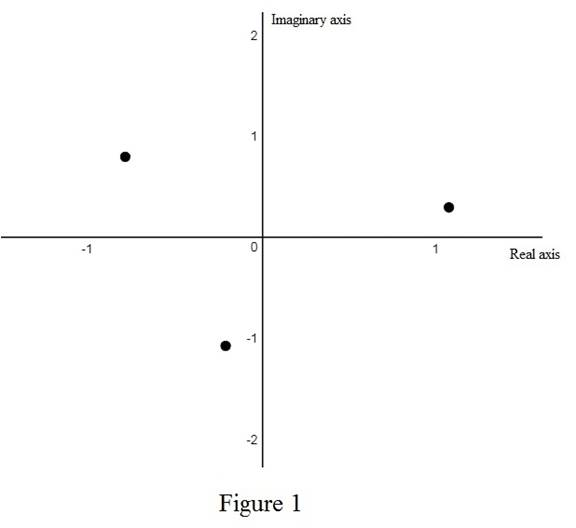# The roots of the cube root of ( 1 + i ) and sketch the roots in the complex plane.### Single Variable Calculus: Concepts...

4th Edition
James Stewart
Publisher: Cengage Learning
ISBN: 9781337687805### Single Variable Calculus: Concepts...

4th Edition
James Stewart
Publisher: Cengage Learning
ISBN: 9781337687805

#### Solutions

Chapter I, Problem 40E
To determine

## To find: The roots of the cube root of (1+i) and sketch the roots in the complex plane.

Expert Solution

The roots of the cube root of (1+i) are wk=216[cos(π4+2kπ3)+isin(π4+2kπ3)] where k=0,1,2.

### Explanation of Solution

Theorem used:

Roots of a complex number:

Let z=r(cosθ+isinθ) and n be a positive integer. Then z has the n distinct nth roots wk=r1n[cos(θ+2kπn)+isin(θ+2kπn)] where k=0,1,2,,n1.

Calculation:

Rewrite the complex number (1+i) in polar form.

The polar form of the complex number z=a+bi is z=r(cosθ+isinθ) where r=|z|=a2+b2  and tanθ=ba.

Consider the complex number (1+i). Here, a=1, b=1 and n=3.

Obtain the argument of the complex number (1+i).

tanθ=11=1

Thus, the argument of the complex number (1+i) is θ=tan1(1)=π4

Obtain the modulus of the complex number (1+i).

r=|1+i|=12+(1)2=2

Thus, the value of r=2.

Therefore, the polar form of the complex number (1+i) is 1+i=(cosπ4+isinπ4).

By the above theorem, the roots of the cube root of (1+i) are wk=(2)13[cos(π4+2kπ3)+isin(π4+2kπ3)]=216[cos(π4+2kπ3)+isin(π4+2kπ3)] where k=0,1,2.

Use online calculator to sketch the roots in the complex plane as shown below in Figure 1.From figure 1, it is observed that all cube root of i form a triangle on complex plane.

### Have a homework question?

Subscribe to bartleby learn! Ask subject matter experts 30 homework questions each month. Plus, you’ll have access to millions of step-by-step textbook answers!